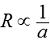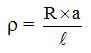# Physics

### Chapter : 1. Electricity

#### Resistivity

ResistivitySo,or...(i)
where ρ (rho) is called resistivity of the material of conductor.
यदि, l = 1 m और a = 1 m2
तब R = ρ ...(ii)
Thus, if we take 1 metre long piece of a substance having a cross-sectional area of 1 meter2, then the resistance of that piece of the substance is called its resistivity.
Resistivity of a substance can also be defined as follows :
The resistance offered by a cube of a substance having side of 1 metre, when current flows perpendicular to the opposite faces, is called its resistivity.
Units of Resistivity
From equation (i), we can writeSo, SI unit of resistivity (ρ)
== ohm.m
Thus, the SI unit of resistivity is ohm. m (or Ω .m).